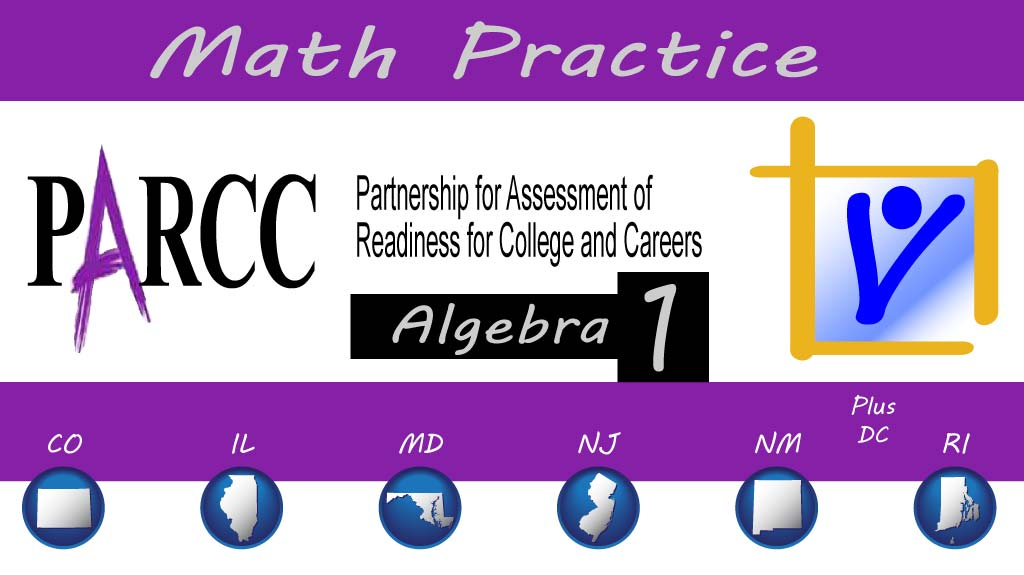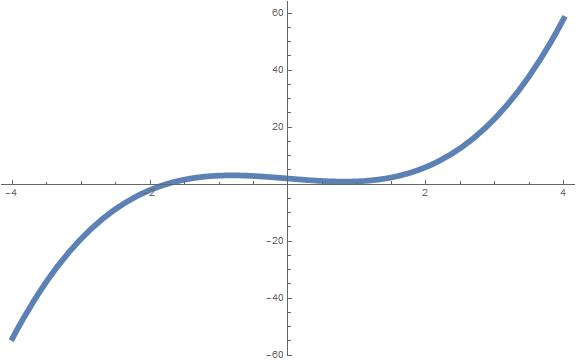Hot Topics

# Algebra 1 PARCC: rewrite polynomial

#### The following dropdown-menu question, explained here in hopes of helping algebra 1 students and their parents in Maryland and Illinois prepare for the PARCC test near the end of this school year, appears on the released version of PARCC’s Spring 2016 test for algebra 1 (#4):The polynomial (2x – 1)(x2 – 2) – x (x2x – 2) can be written in the form ax3 + bx2 + cx + d, where a, b, c, and d are constants.

What are the values of a, b, c, and d?

(Only five choices are available for each:

• –2
• –1
• 0
• 1
• 2)

Correct answer: a = 1, b = 0, c = –2, d = 2.

Common Core Math, High School Algebra, Arithmetic with Polynomials & Rational Expressions:

The question tests students’ understanding of Common Core standards in high school algebra, specifically those under arithmetic with polynomials and rational expressions, which require students to be able to (APR.A) “perform arithmetic operations on polynomials” by (APR.A.1) “understanding that polynomials form a system analogous to the integers, namely, they are closed under the operations of addition, subtraction, and multiplication; add, subtract, and multiply polynomials.”

In this problem, students perform several arithmetic operations on polynomials, including addition, subtraction, and multiplication. The problem does not test the first part of the standard or students’ understanding of these operations in terms of the mathematical significance of those operations.

Solution strategy (there are others)

Multiply the factors and combine like terms.

For the first term, we can use the FOIL rule in a straightforward way, since the two factors are both binomials. For the second term, we can apply the distributive property, also in a straightforward way. To avoid confusion with negative and subtraction signs, I’m just going to distribute the x over the trinomial, keeping the minus sign as a minus sign for now.$(2x-1)(x^2-2) - x(x^2-x-2)$$(2x^3-1x^2-4x+2) - (x^3-x^2-2x)$

At this point, we have a sum of terms in varying powers of x, so we can now distribute the minus sign as a –1 over the three terms of the trinomial.$2x^3-1x^2-4x+2 -x^3+x^2+2x$$x^3-2x+2$

As a result of combining like terms, which means to take the two terms in x3, for instance, and perform the operation specified (2x3 – 1x3 = 1x3); the x2 term vanished, meaning the coefficient became 0.

With that, we have a polynomial in the form ax3 + bx2 + cx + d.

To check your answer, you can plot both polynomials using a computer algebra system like Mathematica. Both polynomials have a function that looks like this:## Analysis of this question and online accessibility

The question is valid in that it tests students’ ability to perform standard arithmetic operations on polynomials.

Guessing is not easy, but the presentation of only five drop-down choices for each of the four coefficients gives this question the feel of four multiple-choice questions, which would tend to promote guessing. It takes longer, though, to guess and check, so most students, I suspect, will just work the problem.

Multiple-choice varieties like this one can be delivered online or on paper, and reliability, validity, and fairness performance should be equivalent, regardless of the delivery mode.

No special accommodation challenges can be identified with this question, so the question is considered fair.

## Resources for further study

Purple Math, developed by Elizabeth Stapel, a math teacher from the St Louis area, has developed several pages dealing with arithmetic operations on polynomials, including pages that show you how to combine like terms, add polynomials, and use the FOIL strategy.

Ron Larson, Laurie Boswell, Timothy D Kanold, Lee Stiff. Algebra 2, Illinois edition. Evanston, Ill.: McDougal Littell, a division of Houghton Mifflin Company, 2008. The book is used in several algebra classes taught in Illinois high schools.

Foerster, Paul A. Algebra and Trigonometry: Functions and Applications, revised edition. Addison-Wesley, 1980, 1984. The book is used in several algebra classes taught in middle and high schools in both Illinois and Maryland.

## Purpose of this series

To help algebra 1 students and their parents prepare for the PARCC test in math, as administered in at least six states, or to just master content on that test, we provide an analysis of every algebra 1 math problem PARCC released in 2016. The series can be found here.

#### About the Author

##### Paul Katula
Paul Katula is the executive editor of the Voxitatis Research Foundation, which publishes this blog. For more information, see the About page.